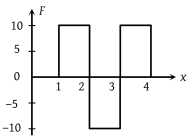Figure shows the F-x graph. Where F is the force applied and x is the distance covered by the body along a straight line path. Given that F is in newton and x in metre, what is the work done ?(1) 10 J

(2) 20 J

(3) 30 J

(4) 40 J